# ILP Part 29 — Nonograms

This is the twenty ninth part of the ILP series. For your convenience you can find other parts in the table of contents in Part 1 – Boolean algebra

Hello! Today we are going to solve nonograms using ILP.

Nonograms are picture logic puzzles in which cells in a grid must be colored or left blank according to numbers at the side of the grid to reveal a hidden picture. Images are usually black and white, however, they can be colored. Below is an example of a nonogram (by wikipedia):We will solve black and white nonogram, so we assume that numbers describe blocks of black fields whit at least one white field between every block.

# Variables

As with most of the riddles we start with defining binary variables representing decisions. Let’s assume that the picture has size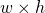(width and height). For every field we define a binary variable meaning whether we fill this field or no: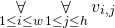We also need to define variables representing top-left blocks’ corners. Let’s introduce the following notation: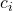means number of blocks in-th column,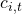means length of-th block in-th column. Analogously,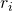means number of block in-th row,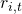means length of-th block in-th row. We define the following binary variables: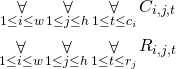Variable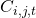means whether-th block in-th column starts (e.g., its top-left field is) in field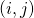. Analogously,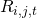means whether-th block in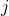-th row starts in field.

After solving the problem we obtain the solution from variables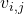. Other variables are used only to specify constraints.

# Constraints

We start with two obvious constraints: number of selected fields in each row and each column must match the blocks’ lengths: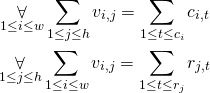Now we need to make sure that in every field there starts at most one block: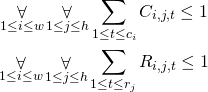Next, we need to make sure that if the block starts in particular field, all fields of this block are selected: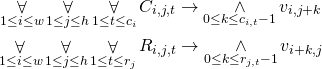When a particular column block starts in field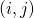(which means that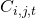is true) then all fields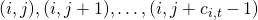must be true so we use conjunction. The same idea goes for row blocks. We also need to make sure that block does not start close to the boundary: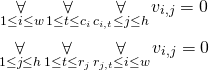Finally, we need to take care of blocks ordering. We need to make sure that block occur in proper order. We use the following idea: if some column block starts in fieldwhich means that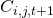is true, previous block must start in some field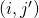where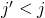. So the sum of variables indicating start of the block must be true. The following constraints take care of this: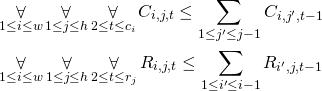Ifis true then sum of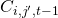must be true in order to fulfill the constraint. On the other hand, ifis false, then sum ofcan have any value. The same goes for rows.

# Summary

As we can see implementation is rather easy. The most difficult part is related to ordering the blocks, the rest is pretty straightforward. Next time we will solve sudoku.